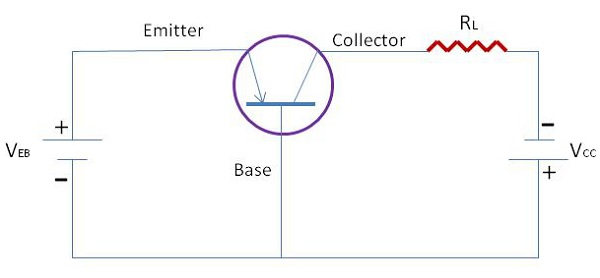# Configuration of Transistors

When a transistor is connected in a circuit, four terminals or leads or legs are required, two both for input and output. As we know that transistors have only 3 terminals, this situation can be overcome by making one of the terminal common for both input and output section. Accordingly, a transistor can be connected in three configurations as follows −

• Common Base Configuration
• Common Emitter Configuration
• Common Collector Configuration

Following are some important points to note about transistor operation.

• A transistor can be operated in three regions namely active, saturation, and cutoff region.

• A transistor when used in the active region, the base-emitter junction is forward biased and the collector-base junction is reverse biased.

• A transistor when used in the saturation region, the base-emitter junction is forward biased and the collector-base junction is also forward biased.

• A transistor when used in the cut-off region, both the base-emitter junction and collector-base junction are reverse biased.

## Comparison of Transistor Configuration

The following table shows the comparison of transistor configuration.

Characteristics Common Emitter Common Base Common Collector
Current Gain High No Considerable
Applications Audio frequency High frequency Impedance matching
Input Resistance Low Low Very high
Output Resistance High Very high Low
Voltage Gain Approx. 500 Approx. 150 Less than 1

Low source voltage Temperature dependency
High voltage gain Lower power dissipation
Smaller in size Low input impedance

## Current Amplification Factor (α)

The ratio of change in the collector current to the change in the emitter current at constant collector to base voltage Vcb is known as current amplification factor ‘α’. It can be expressed as

$\alpha = \frac{\Delta I_C}{\Delta I_B}$ at Constant VCB

It is clear that current amplification factor is less than unity and it is inversely proportional to the base current considered that the base is lightly doped and thin.

## Base Current Amplification Factor (β)

It is the ratio of change in collector current to the change in base current. A small variation in base current results in a very large change in collector current. Therefore, the transistor is able to attain current gain. It can be expressed as

$$\beta = \frac{\Delta I_C}{\Delta I_B}$$

## Transistor as an Amplifier

The following figure shows that a load resistor (RL) is in series with the collector supply voltage (Vcc). A small voltage change ΔVi between the emitter and the base causes a relatively large emitter-current change ΔIE.We define by the symbol ‘a’ - the fraction of this current change - which is collected and passes through RL. The change in output voltage across the load resistor ΔVo = a’RL ΔIE may be many times the change in input voltage ΔVI. Under these circumstances, the voltage amplification A == VO/ΔVI will be greater than unity and the transistor acts as an amplifier.